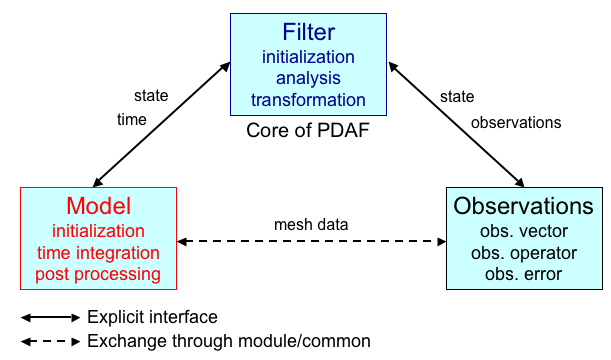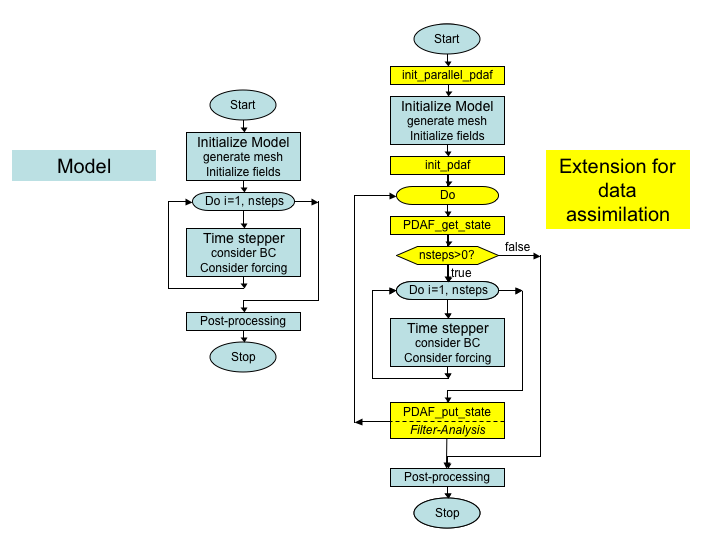Version 14 (modified by lnerger, 10 years ago) (diff)

--

# General Implementation Concept of PDAF

## Logical separation of the assimilation system

PDAF bases on the localization separation of the assimilation system in 3 parts. These are depicted in figure 1.Figure 1: Logical structure of an assimilation system.

The parts of the assimilation system are

• Model:
The numerical model provides the initialization and integration of all model fields. It defines the dynamics of the system that is simulated.
• Observations:
The observations of the system provide additional information.
• Filter:
The filter algorithms combine the model and observational information.

Generally, all three components are independent. In particular, the filters are implemented in the core part of PDAF. To combine the model and observational information one has to define the relation of the observations to the models fields (For example, model fields might be directly observed or the observed quantities are more complex functions of the model fields? In addition, the observations might be available on grid points. If not, interpolation is required.) In addition one has to define the relation of the state vector that is considered in the filter algorithms to the model fields. These relations are defined in separate routines that are supplied to the assimilation system by the user. These routines are called through a well-defined standard interface. To ease the implementation complexity, these user-defined routines can be implemented like routines of the model code. Thus, if a user has experience with the model, it should be rather easy to extend it by the routines required for the assimilation system.

## Online and offline assimilation systems

There are two possibilities to build a data assimilation system

1. Offline mode: The model is executed separately from the assimilation/filter code. Output files from the model are used as inputs for the assimilation program.
2. Online mode: The model code is extended by calls to PDAF core routines. A single executable is compiled. While running this single executable the necessary ensemble integrations and the actual assimilation is performed.

PDAF supports both the online and offline modes. Generally, we recommend to use the online mode because it is more efficient on parallel computers. However, the required coding is simpler for the offline than the online mode.

## Online mode: Attaching PDAF to a model

Here we describe the extensions of the model code for the online mode of PDAF.

The assimilation system is built by adding call to PDAF-routines to the general part of the model code. As only minimal changes to the model code are required, we refer to this as "attaching" PDAF to the model.

The general concept is depicted in figure 2. The left hand side shows a typical abstract structure of a numerical model. When the program is executed, the following steps are performed:

1. The model is initialized. Thus arrays for the model fields are allocated and filled with initial fields. Thus, the model grid is build up virtually in the program.
2. After the initialization the time stepping loop is performed. Here the model fields are propagated through time.
3. When the integration of the model fields is completed after a defined number of time steps, various post-processing operations are performed. Then the program stops.Figure 2: (left) Generic structure of a model code, (right) extension for data assimilation with PDAF

The right hand side of Figure 2 shows the extensions required for the assimilation system (marked yellow):

• Close to the start of the model code the routine `init_parallel_pdaf` as added to the code. If the model itself is parallelized the correct location is directly after the initialization of the parallelization in the model code. `init_parallel_pdaf` creates the parallel environment that allows to perform several time stepping loops at the same time.
• After the initialization part of the model, a routines `init_pdaf` is added. In this routine, parameters for PDAF can be defined and then the core initialization routine PDAF_init is called. This core routine also initializes the array of ensemble states.
• In order to allow for the integration of the state ensemble an unconditional loop is added around the time stepping loop of the model. This will allow to compute the time stepping loop multiple time during the model integration. PDAF provide an exit-flag for this loop. (There are some conditions, under which this external loop is not required. Some note on this are given further below.)
• Inside the external loop the PDAF core routine `PDAF_get_state` is added to the code. This routine initializes model fields form the array of ensemble states and initialized the number of time step that have to be computed and ensured that the ensemble integration is performed correctly.
• At the end of the external loop, the PDAF core routine `PDAF_put_state` is added to the model code. This routine write the propagated model fields back into a state vector of the ensemble array. Also it checks whether the ensemble integration is complete. If not, the next ensemble member will be integrated. If the ensemble integration is complete, the analysis step (i.e. the actual assimilation of the observations) is computed.

With the implementation strategy of PDAF, four routines and the external loop have to be added to the model code. While this looks like a large change in figure 2, this change does actually only affect the general part of the model code. In addition, the amount of source code of the numerical model will be much longer than the addition for the data assimilation system.

## Remarks on the implementation concept

• The implementation concept of PDAF does not require that the time stepping part of the model is implemented as a subroutine. Instead calls to subroutines that control of the ensemble integration are added to the model code before and after the code parts performing the time stepping. If the time stepping part is implemented as a subroutine, this subroutine can be called in between the additional routines.
• Depending on the parallelization, there can be cases in which the model has to jump back in time and cases in which the time always moves forward:
• Jumping back in time will be required if the number of model tasks used to evolve the ensemble states is smaller than the number of ensemble members. In this case a model task has integrate more than one model state and will have to jump back in time after the integration of each ensemble member.
• If there are as many model tasks as ensemble members, the model time always moves forward. In this case, one can implement PDAF also without the external ensemble loop. That is, one can add calls to `PDAF_get_state` and `PDAF_put_state` directly into the code of the model's time stepping loop. This strategy might also be called for, if a model uses staggered loops (like a loop over minutes inside a loop over hours).
• Model-specific operations like the initialization of the array of ensemble states in `PDAF_init` are actually performed by user-supplied routines. These routines are called through the standard interface of `PDAF`. Details on the interface and the required routines are given on the pages describing the implementation steps.
• The assimilation system is controlled by the user-supplied routines that are called through PDAF. With this strategy, the assimilation program is essentially driven by the model part of the program. Thus, logically the model is not a sub-component of the assimilation system, but the implementation with PDAF results in a model extended for data assimilation.
• The user-supplied routines can be implemented analogously to the model code. For example, if the model is writting using Fortran common blocks or modules of the model code, these can be used to implement the user-supplied routines, too. This simplifies the implementation of the user-supplied routines knowing about the particularities of their model.